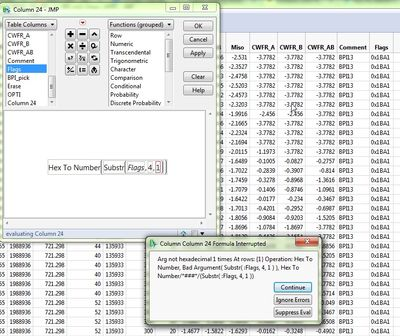Choose Language Hide Translation Bar
Highlighted

## how to extract certain bits from a string hex column to number ?

Hello, Gurus,

I have a very simple question on how to extract certain bits from a string hex column(named Flags) to numbers. My column values will be like "0x1BA1". I would like to extract the bits 8 and 9 values and convert them to a value of 3 into my new column. Since I don't know the binary operation in jmp, I tried to extract B into 11 first by doing the following

Char To Hex(Munger(:Flags, 4, 1)).

It gives me a character column of 42. I tried to put a "integer" in to the formula

Char To Hex((Munger(:Flags, 4, 1)), "integer")

But it wouldn't recognize it. I'm using jmp11.2.1.

After extracting B, I'll use module(module(value,8),4) to get the lower 2 bits.

If there's an easy way to extract binary bits, that will be even better.

Thank you,

Jane

1 ACCEPTED SOLUTION

Accepted Solutions
Highlighted

## Re: how to extract certain bits from a string hex column to number ?

As Craige pointed out (thanks, I was not aware), JMP 11 requires an even number of hex characters.

Try this formula for single character substrings:

Hex To Number("0" || Substr(Flags, 4, 1));

6 REPLIES 6
Highlighted

## Re: how to extract certain bits from a string hex column to number ?

There is a function Hex to Number() that converts hexadecimal numbers (as strings) to decimal numbers.

Example:

hex = "0x1BA1";

// Hex to decimal

value = Hex To Number(Substr(hex, 4, 1));

Show(value);

// decimal to binary

bin = "";

While(value,

bin = Char(Modulo(Num(value), 2)) || bin;

value = Floor(value / 2);

);

Show(bin);

// Reverse last step for confirmation (or just for fun)

value2 = Sum(2 ^ (Loc(Reverse(Words(bin, "")), "1") - 1));

Show(value2);

Highlighted

## Re: how to extract certain bits from a string hex column to number ?

before JMP 12 you'll need an even number of hex characters:

Hex To Number( "0B" )

11

Craige
Highlighted

## Re: how to extract certain bits from a string hex column to number ?

Hi, Craig,

Thank you. The magic "0" in front of "B" is the key.

Jane

Highlighted

## Re: how to extract certain bits from a string hex column to number ?

Hello, MS,

I have trouble with these "Hex to .." command. They don't work on my case. Here's the screen copy. I don't know what could be wrong.

Thank you,

JaneHighlighted

## Re: how to extract certain bits from a string hex column to number ?

As Craige pointed out (thanks, I was not aware), JMP 11 requires an even number of hex characters.

Try this formula for single character substrings:

Hex To Number("0" || Substr(Flags, 4, 1));

Highlighted

## Re: how to extract certain bits from a string hex column to number ?

Thank you, MS.

I tried the concat "0" and it works.

Jane

Article Labels

There are no labels assigned to this post.